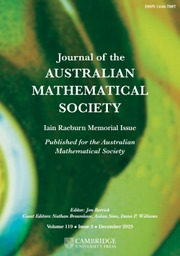Home
Hostname: page-component-8bbf57454-9s44l Total loading time: 0.216 Render date: 2022-01-21T23:22:21.996Z Has data issue: true Feature Flags: { "shouldUseShareProductTool": true, "shouldUseHypothesis": true, "isUnsiloEnabled": true, "metricsAbstractViews": false, "figures": true, "newCiteModal": false, "newCitedByModal": true, "newEcommerce": true, "newUsageEvents": true }Journal of the Australian Mathematical Society

Abstract

We characterize the second order subexponentiality of an infinitely divisible distribution on the real line under an exponential moment assumption. We investigate the asymptotic behaviour of the difference between the tails of an infinitely divisible distribution and its Lévy measure. Moreover, we study the second order asymptotic behaviour of the tail of the$t$th convolution power of an infinitely divisible distribution. The density version for a self-decomposable distribution on the real line without an exponential moment assumption is also given. Finally, the regularly varying case for a self-decomposable distribution on the half line is discussed.

MSC classification

Type
Research Article
© 2020 Australian Mathematical Publishing Association Inc.

Access options

Get access to the full version of this content by using one of the access options below. (Log in options will check for institutional or personal access. Content may require purchase if you do not have access.)

References

Asmussen, S., Foss, S. and Korshunov, D., ‘Asymptotics for sums of random variables with local subexponential behaviour’, J. Theoret. Probab. 16 (2003), 489518.CrossRefGoogle Scholar
Baltrunas, A., ‘Second order behaviour of ruin probabilities’, Scand. Actuar. J. 2 (1999), 120133.CrossRefGoogle Scholar
Bingham, N. H., Goldie, C. M. and Teugels, J. L., Regular Variation (Cambridge University Press, Cambridge, 1984).Google Scholar
Bondesson, L., ‘On the Lévy measure of the lognormal and the logcauchy distributions’, Methodol. Comput. Appl. Probab. 4 (2002), 243256.CrossRefGoogle Scholar
Chover, J., Ney, P. and Wainger, S., ‘Functions of probability measures’, J. Anal. Math. 26 (1973), 255302.CrossRefGoogle Scholar
Embrechts, P., Goldie, C. M. and Veraverbeke, N., ‘Subexponentiality and infinite divisibility’, Z. Wahrscheinlichkeitstheorie verw. Geb. 49 (1979), 335347.CrossRefGoogle Scholar
Foss, S., Korshunov, D. and Zachary, S., An Introduction to Heavy-Tailed and Subexponential Distributions, 2nd edn, Springer Series in Operations Research and Financial Engineering (Springer, New York, 2013).CrossRefGoogle Scholar
Geluk, J. L., ‘Second order tail behaviour of a subordinated probability distribution’, Stochastic Process. Appl. 40 (1992), 325337.CrossRefGoogle Scholar
Geluk, J. L. and Pakes, A. G., ‘Second order subexponential distributions’, J. Aust. Math. Soc. 51 (1991), 7387.Google Scholar
Jiang, T., Wang, Y., Cui, Z. and Chen, Y., ‘On the almost decrease of a subexponential density’, Statist. Probab. Lett. 153 (2019), 7179.CrossRefGoogle Scholar
Klüppelberg, C., ‘Subexponential distributions and integrated tails’, J. Appl. Probab. 25 (1988), 132141.CrossRefGoogle Scholar
Klüppelberg, C., ‘Subexponential distributions and characterizations of related classes’, Probab. Theory Related Fields 82 (1989), 259269.CrossRefGoogle Scholar
Lin, J., ‘Second order subexponential distributions with finite mean and their applications to subordinated distributions’, J. Theoret. Probab. 25 (2012), 834853.CrossRefGoogle Scholar
Omey, E. and Willekens, E., ‘Second order behaviour of the tail of a subordinated probability distribution’, Stochastic Process. Appl. 21 (1986), 339353.CrossRefGoogle Scholar
Omey, E. and Willekens, E., ‘Second-order behaviour of distributions subordinate to a distribution with finite mean’, Comm. Statist. Stochastic Models 3 (1987), 311342.CrossRefGoogle Scholar
Pakes, A. G., ‘Convolution equivalence and infinite divisibility’, J. Appl. Probab. 41 (2004), 407424.CrossRefGoogle Scholar
Sato, K., Lévy Processes and Infinitely Divisible Distributions, Cambridge Studies in Advanced Mathematics, 68 (Cambridge University Press, Cambridge, 2013).Google Scholar
Shimura, T. and Watanabe, T., ‘Subexponential densities of compound Poisson sums and the supremum of a random walk’, Preprint, 2020, arXiv:2001.11362.Google Scholar
Steutel, F. W. and van Harn, K., Infinite Divisibility of Probability Distributions on the Real Line, Pure and Applied Mathematics: A Series of Monographs and Textbooks, 259 (Marcel Dekker, New York–Basel, 2004).Google Scholar
Watanabe, T., ‘Convolution equivalence and distributions of random sums’, Probab. Theory Related Fields 142 (2008), 367397.CrossRefGoogle Scholar
Watanabe, T., ‘Subexponential densities of infinitely divisible distributions on the half line’, Lith. Math. J. (2020), to appear.Google Scholar
Watanabe, T. and Yamamuro, K., ‘Local subexponentiality of infinitely divisible distributions’, J. Math-for-Ind. 1 (2009), 8190.Google Scholar
Watanabe, T. and Yamamuro, K., ‘Local subexponentiality and self-decomposability’, J. Theoret. Probab. 23 (2010), 10391067.CrossRefGoogle Scholar
2
Cited by

Send article to Kindle

Note you can select to send to either the @free.kindle.com or @kindle.com variations. ‘@free.kindle.com’ emails are free but can only be sent to your device when it is connected to wi-fi. ‘@kindle.com’ emails can be delivered even when you are not connected to wi-fi, but note that service fees apply.

Find out more about the Kindle Personal Document Service.

SECOND ORDER SUBEXPONENTIALITY AND INFINITE DIVISIBILITY
Available formats
×

Send article to Dropbox

To send this article to your Dropbox account, please select one or more formats and confirm that you agree to abide by our usage policies. If this is the first time you use this feature, you will be asked to authorise Cambridge Core to connect with your <service> account. Find out more about sending content to Dropbox.

SECOND ORDER SUBEXPONENTIALITY AND INFINITE DIVISIBILITY
Available formats
×

To send this article to your Google Drive account, please select one or more formats and confirm that you agree to abide by our usage policies. If this is the first time you use this feature, you will be asked to authorise Cambridge Core to connect with your <service> account. Find out more about sending content to Google Drive.

SECOND ORDER SUBEXPONENTIALITY AND INFINITE DIVISIBILITY
Available formats
×
×